#### Factorising a 2x2 non-singular non-negative matrix

Input: a non-singular matrix A =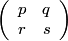, p, q, r, s ≥ 0.
A non-negative matrix B =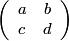is called row-balanced if (a < c & b > d) or (c < a & d > b).
With L =and R =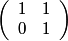, we express A uniquely as a product of positive powers of L and R, followed by a row-balanced B.
We exclude A =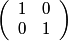and A =.
With Ua =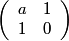, we note that Ua0··· Ua2n = Ra0La1··· Ra2nU0 and that Ua0··· Ua2n+1 = Ra0La1··· La2n+1I2.

The number of terms L and R in the factorisation is returned.

We remark that if A is a positive unimodular matrix, the factorisation cannot consist wholly of powers of R or powers of L.

If rp and sq, we take m = min([r/p], [s/q]) if p > 0 and q > 0, m = [r/p] if q = 0 and m = [s/q] if p = 0 and use the identity= LmB.

whereas if pr and qs, we take m = min([p/r], [q/s]), if r > 0 and s > 0, m = [p/r] if s = 0 and m = [q/s] if r = 0 and use the identity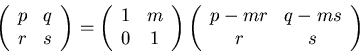= RmB.

B is a non-negative matrix whose entries are not greater than before and for which at least one entry has strictly decreased.

(See On continued fractions and finite automata, George N. Raney, Math. Annalen, 206, 265-283 (1973).)

Enter p (≥ 0):
Enter q (≥ 0):
Enter r (≥ 0):
Enter s (≥ 0):